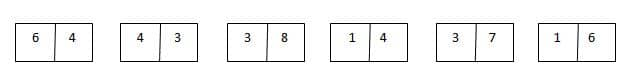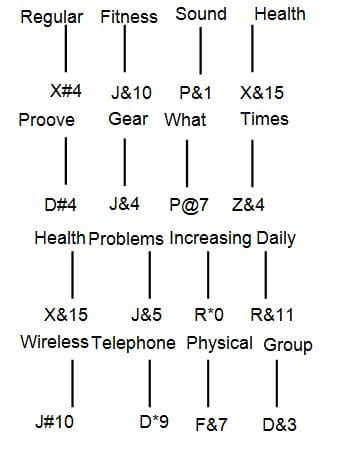# gkrecall

We brings you daily Current affairs, daily Current Affairs Quiz, weekly Current Affairs, weekly Current Affairs Quiz to enhance your preparation for upcoming exams. We also provide articles related to quant, reasoning, English, ssc etc .

## Breaking

We bring you the complete and important daily Reasoning  to achieve more marks in Banking, Insurance, UPSC, SSC, CLAT, Railways and all other competitive Exams. We prepare it based on our daily current affairs.Hope you like it.

START QUIZ

Directions (1 – 5): Study the given information carefully and answer the given questions:
An input-output is given in different steps. Some mathematical operations are done in each step. No mathematical operation is repeated in next step.

1.What is the multiplication of all the numbers of step 2?
(a) 8
(b) 6
(c) 12
(d) 15
(e) None of these

2.Which is the largest number obtained in step 1?

(a) 188
(b) 244
(c) 249
(d) 289
(e) 250

3.If the third number of all the boxes is halved and then added the half numbers, what is the result in step 1?

(a) 10.5
(b) 12.5
(c) 8.5
(d) 11.5
(e) None of these

4.What is the sum of numbers in step 3?

(a) 0.66
(b) 0.74
(c) 1.83
(d) 1.66
(e) 1.35

5.Which is the following number obtained in last step?

(a) 1.07
(b) 1.77
(c) 1.67
(d) 1.17
(e) None of these

Solution-

In first step:
Multiply 1st and 2nd number of box 1 and 4 respectively: 5 × 4 = 20
Multiply 2nd and 1st number of box 1 and 4 respectively: 3 × 2 = 6
Multiply 1st and 2nd number of box 2 and 5 respectively: 7 × 3 = 21
Multiply 2nd and 1st number of box 2 and 5 respectively: 2 × 4 = 8
Multiply 1st and 2nd number of box 3 and 6 respectively: 6 × 7 = 42
Multiply 2nd and 1st number of box 3 and 6 respectively: 2 × 3 = 6In second step:
Add 1st and 3rd number of box 1 and subtract it from 2nd number of box 1 = 6 + 2 = 8, 8 – 0 = 8
Add 1st and 3rd number of box 2 and subtract it from 2nd number of box 2 = 8 + 2 = 10, 10 – 1 = 9
Add 1st and 3rd number of box 3 and subtract it from 2nd number of box 3 = 6 + 4 = 10, 10 – 2 = 8

In third step:
Divide 2nd number from 1st number = 9 ÷ 8 = 1.12
Divide 3rd number from 2nd number = 8 ÷ 9 = 0.89
In fourth step:
Subtract both the numbers = 1.12 – 0.89 = 0.23In first step:
Multiply 1st and 2nd number of box 1 and 4 respectively: 6 × 4 = 24
Multiply 2nd and 1st number of box 1 and 4 respectively: 4 × 1 = 4
Multiply 1st and 2nd number of box 2 and 5 respectively: 4 × 7 = 28
Multiply 2nd and 1st number of box 2 and 5 respectively: 3 × 3 = 9
Multiply 1st and 2nd number of box 3 and 6 respectively: 3 × 6 = 18
Multiply 2nd and 1st number of box 3 and 6 respectively: 8 × 1 = 8
In second step:
Add 1st and 3rd number of box 1 and subtract it from 2nd number of box 1 = 4 + 2 = 6, 6 – 4 = 2
Add 1st and 3rd number of box 2 and subtract it from 2nd number of box 2 = 9 + 2 = 11, 11 – 1 = 3
Add 1st and 3rd number of box 3 and subtract it from 2nd number of box 3 = 8 + 1 = 9, 9 – 8 = 1
In third step:
Divide 2nd number from 1st number = 3 ÷ 2 = 1.5
Divide 3rd number from 2nd number = 1 ÷ 3 = 0.33

In fourth step:
Subtract both the numbers = 1.5 – 0.33 = 1.17
Direction (6 – 10): Read the following information carefully and answer the questions that follow:
In a certain code language,
“Regular fitness sound health” is coded as “J&10 X&15 X#4 P&1”
“Proove gear what times” is coded as “P@7 D#4 Z&4 J&4”
“Health problems increasing daily” is coded as “J&5 R&11 X&15 R*0”
“Wireless telephone physical group” is coded as “D&3 J#10 D*9 F&7”
6.What is the code for word ‘solution’?
(a) R@2
(b) R#1
(c) R*0
(d) J!17
(e) None of these
7.Which of the following is the code for ‘phone call’?
(a) R&5 B@12
(b) R@6 B!12
(c) D*6 B!11
(d) D&6 B@11
(e) None of these
8.‘B#1’ is the code for which of the following?
(a) Spherical
(b) Physical
(c) Education
(d) Important
(e) Cannot be determined
9.What is the code for ‘delete data’?
(a) K#12 B#14
(b) K@15 B*14
(c) J#15 B@5
(d) J#15 B&19
(e) None of these
10.What is the code for ‘parenting’?
(a) R!12
(b) R#13
(c) E%12
(d) R*13
(e) J@12
Solution-
Logic:
Number – Subtract the position of 2nd alphabet from right end with 2nd alphabet from left end.
Symbol – Symbol is coded according to number of vowels in the word:
# – 3
@ – 1
* – 4
! – 5
& – 2
Alphabet – Position of 3rd alphabet from right end. Add same number to it to get the alphabet
For example – Wireless
Number – Position of ‘I’ in English alphabet = 9
Position of ‘S’ in English alphabet = 19
Subtract 19 – 9 = 10
Symbol – Number of vowels in ‘wireless’ = 3
Symbol – #
Alphabet – 3rd alphabet from right end = O
Position of ‘E’ = 5
5 + 5 = 10
Alphabet – J
Hence, ‘wireless’ is coded as ‘J#10’.